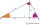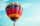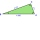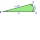# Right triangle calculator

Please enter two properties of the right triangle

Use symbols: a, b, c, A, B, h, T, p, r, R

The right triangle calculators compute angles, sides (adjacent, opposite, hypotenuse) and area of any right-angled triangle and use it in the real world. Two independent properties entirely determine any right-angled triangle. The calculator provides a step-by-step explanation for each calculation.

A right triangle is a kind of triangle that has one angle that measures C=90°. In a Right triangle, the side c that is opposite of the C=90° angle, is the longest side of the triangle and is called the hypotenuse. The variables a, b are the lengths of the shorter sides, also called legs or arms. Variables for angles are A, B, or α (alpha) and β (beta). Variable h refers to the altitude(height) of the triangle, which is the length from the vertex C to the hypotenuse of the triangle.

Examples for right triangle calculation:

## A right triangle in word problems in mathematics:

• Triangle P2Can a triangle have two right angles?
• Vector 7Given vector OA(12,16) and vector OB(4,1). Find vector AB and vector |A|.
• Height of right RTThe right triangle ABC has a hypotenuse c 9 cm long and a part of the hypotenuse cb = 3 cm. How long is the height of this right triangle?
• Cable carCable car rises at an angle 45° and connects the upper and lower station with an altitude difference of 744 m. How long is "endless" tow rope?
• Spruce heightHow tall was spruce that was cut at an altitude of 8m above the ground and the top landed at a distance of 15m from the heel of the tree?
• Altitude angleIn complete winds-free weather, the balloon took off and remained standing exactly above the place from which it took off. It is 250 meters away from us. How high did the balloon fly when we see it at an altitude angle of 25°?
• BisectorsAs shown, in △ ABC, ∠C = 90°, AD bisects ∠BAC, DE⊥AB to E, BE = 2, BC = 6. Find the perimeter of triangle △ BDE.
• In the 18In the right triangle ABC, The hypotenuse AB = 15 cm, and B = 25 degree. How long is BC to the nearest centimetre?
• HeightIs it true that the height is less or equal to half of the hypotenuse in any right triangle?
• Right triangleRight triangle legs has lengths 630 mm and 411 dm. Calculate the area of this triangle.
• Height 2Calculate the height of the equilateral triangle with side 27.The ladder has a length 3.5 meters. He is leaning against the wall so that his bottom end is 2 meters away from the wall. Determine the height of the ladder.
• Euclid2In the right triangle ABC with a right angle at C is given side a=29 and height v=17. Calculate the perimeter of the triangle.
• RT triangle and heightCalculate the remaining sides of the right triangle if we know side b = 4 cm long and height to side c h = 2.4 cm.
• Area of RT 2Calculate the area of right triangle whose legs have a length 5.8 cm and 5.8 cm.